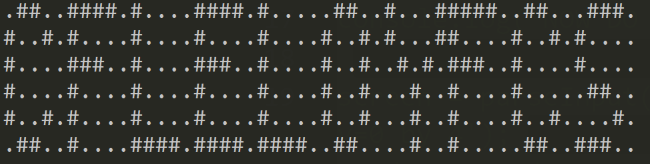Yes - I'm falling behind, but let's just pretend today is still the 8th. Day 8 was a difficult one (I feel like I'm saying that more and more) as it required working with 2D arrays, something I always have trouble with. Specifically - you needed to represent a 2D array of cells on a screen. You then had to take input in the form of:

• rect AxB - which would turn on lights in a rectangle A wide and B high in the upper left corner of the screen.
• rotate row y=A by B - which "rotates" lights in row A B steps lower. You have to handle wrapping too as a light that 'falls off' the bottom then returns to the top.
• rotate column x=A by B - same concept, but in a column.

The answer to this puzzle would simply be the number of lit lights.

The rect part was trivial, but the rotation took me forever. I figured out that given an set of values like so:

ABCDE

and wanting to rotate by, let's say 2, you could create a new set by starting on the third letter and selecting from the original set until you get to the end, and then wrap around, so:

CDEAB

As I've said many times before, using their sample input/output was a huge help. In this case, it helped so much that when I switched from the sample input to the real input, my code returned the right answer immediately!

(I have to admit though, this one was so difficult I contemplated cheating. When you enter a numeric answer in Advent of Code, it usually tells if it is too high or too low. So in theory, I could have gotten the max number of lights and then began guessing by supplying a number half-way through the range and just narrowing it down.)

Here is my solution:

``````
//screen size
let width = 50;
let height = 6;
let screen = seedScreen(width,height);

renderScreen(screen, width, height);
/*
let input = 'rect 3x2';
screen = parseInput(screen, input);
renderScreen(screen, width, height);

console.log('NOW DO COL');
screen = parseInput(screen, 'rotate column x=1 by 1');
renderScreen(screen, width, height);

console.log('NOW DO RECT');

screen = parseInput(screen, 'rotate row y=0 by 4');
renderScreen(screen, width, height);

console.log('NOW DO COL');
screen = parseInput(screen, 'rotate column x=1 by 1');
renderScreen(screen, width, height);
*/

const fs = require('fs');

let good = 0;
let lines = input.split('\n');
lines.forEach(function(line) {
screen = parseInput(screen, line);
renderScreen(screen, width, height);
});

let total = getTotal(screen);
console.log(total);

function getTotal(s) {
let total = 0;
for(let x=0;x<s.length;x++) {
for(let y=0;y<s[x].length;y++) {
if(s[x][y] === "#") total++;
}
}
}

function parseInput(screen, i) {
if(i.indexOf('rect') === 0) {
let dim = i.split(' ');
let width = dim.split('x');
let height = dim.split('x');
return drawRect(screen, width, height);
}

//	rotate row y=A by B
if(i.indexOf('rotate row') === 0) {
let dim = i.split(' row y=');
let row = Number(dim.split(' by '));
let offset = Number(dim.split(' by '));
return rotateRow(screen, width, height, row, offset);
}

//	otate column x=1 by 1
if(i.indexOf('rotate column') === 0) {
let dim = i.split(' column x=');
let col = Number(dim.split(' by '));
let offset = Number(dim.split(' by '));
return rotateColumn(screen, width, height, col, offset);
}

throw('Unknown command: '+i);
}

function rotateColumn(s, w, h, c, o) {
//##.  => .## (1)
//##. => #.# (2)
let originalCol = s[c];
//	console.log('offset is '+o);
//	console.log('origCol='+originalCol.join(''));
let newCol = [];
for(let x=0;x<originalCol.length;x++) {
let thisVal = originalCol[x];
let newPos = x + o;
//		console.log('thisVal='+thisVal+' newPos='+newPos);
if(newPos >= originalCol.length) newPos =  newPos - originalCol.length;
//		console.log(' newPos='+newPos);
newCol[newPos] = thisVal;
}
//	console.log('newCol='+newCol);
s[c] = newCol;
return s;
}

function rotateRow(s, w, h, r, o) {
/*
so given ##00# and offset of 2,
we make a new list starting at 2.
#00##
*/
let originalRow = [];
for(let x=0;x<w;x++) {
for(let i=0;i<h;i++) {
if(i === r) {
originalRow.push(s[x][i]);
}
}
}
//	console.log('or: '+originalRow.join('')+'-');
let newRow = [];
let done = 0;
//because:
o++;
for(let x = o; x < o+originalRow.length; x++) {
let pos = x;
//		console.log('trying to set '+pos);
if(pos > w) pos = pos-w;
//		console.log('really '+pos);
//		newRow.push(originalRow[x]);
//console.log('val to set is '+originalRow[done]);
newRow[pos-1] = originalRow[done];
done++;
//		console.log('new Row len is now '+newRow.length);
}
//	console.log('newRow: '+newRow.join(''));
for(let x=0;x<w;x++) {
for(let i=0;i<h;i++) {
if(i === r) {
s[x][i] = newRow[x];
}
}
}

return s;

}

function drawRect(s,w,h) {
console.log('going to draw '+w+','+h);
for(let i=0;i<w;i++) {
for(let x=0;x<h;x++) {
s[i][x] = "#";
}
}
return s;
}

function seedScreen(w,h) {
let screen = [];
for(let i=0;i<w;i++) {
for(let x=0;x<h;x++) {
//console.log('setting s['+i+']['+x+']');
if(!screen[i]) screen[i] = [];
screen[i][x] = ".";
}
}
return screen;
}

function renderScreen(s,width,height) {
var result = '';
for(let x=0;x<height;x++) {
for(let i=0;i<width;i++) {
result += s[i][x];
}
result += '\n';
}
console.log(result);
}
``````

Notice the `renderScreen` function. I built this to help me debug and it was a fortuitous decision. I noticed when I rendered the 'real' input, the output was a set of letters. Part two to the puzzle was to simply input those letters. Here is what my output looked like:You can find my repo of solutions here: https://github.com/cfjedimaster/adventofcode